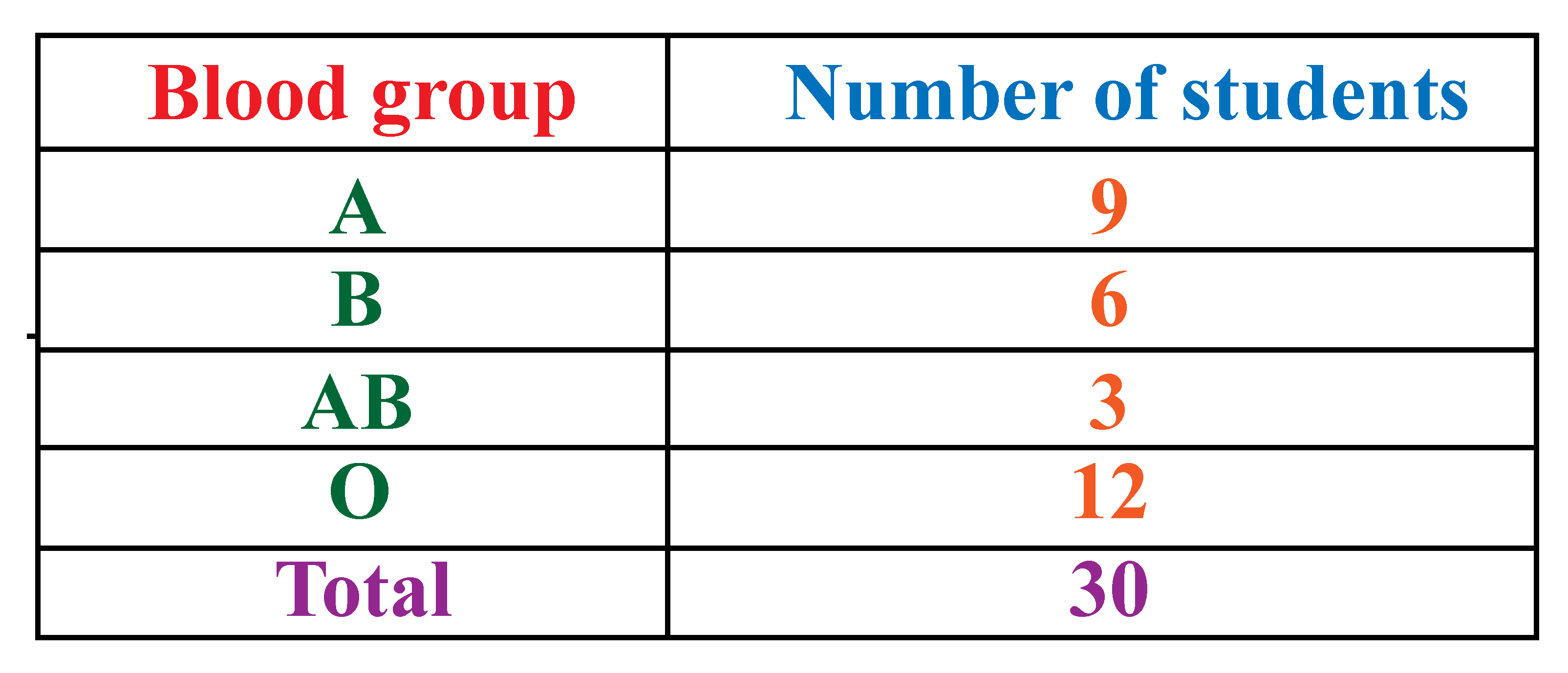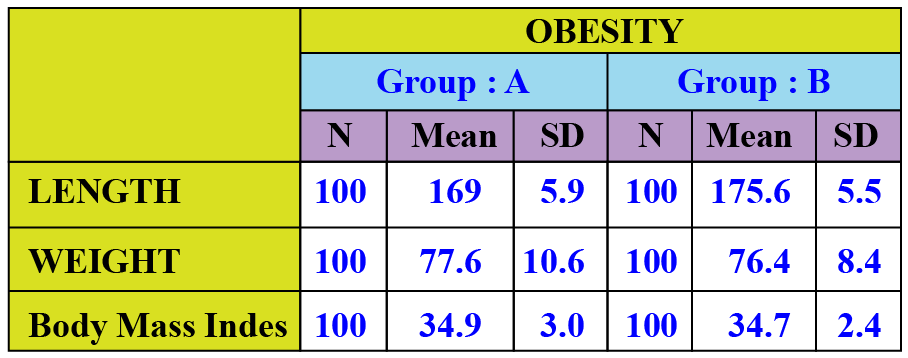# Summary statistics

Summary statistics

Summary statistics helps us summarize statistical information.

Let's consider an example to understand this better.

A school conducted a blood donation camp.

The blood groups of 30 students were recorded as follows.We can represent this data in a tabular form.This table is known as a frequency distribution table.

You can observe that all the collected data is organized under two columns.

This makes it easy for us to understand the given information.

Thus, summary statistics condenses the data to a simpler form so that it is easy for us to observe its features at a glance.

## Lesson Plan

 1 Summary Statistics 2 Challenging Questions on Summary Statistics 3 Important Notes on Summary Statistics 4 Solved Examples on Summary Statistics 5 Interactive Questions on Summary Statistics

## Summary Statistics

Let us first understand the meaning of summary statistics.

Definition of Summary Statistics: Summary statistics is a part of descriptive statistics that summarizes and provides the gist of the information about the sample data.

Summary statistics deals with summarizing statistical information.

This indicates that we can efficiently use summary statistics to quickly get the gist of the information.

Statistics generally deals with the presentation of information quantitatively or visually.

"Summary statistics" is a part of descriptive statistics.

Descriptive statistics deals with the collection, organization, summaries, and presentation of data.

## What Is a Summary Statistics Table?

Big data related to population, economy, stock prices, and unemployment needs to be summarized systematically to interpret it correctly.

It is usually done using a summary statistics table.

The summary table is a visual representation that summarizes statistical information about the data in a tabular form.Here are a few summary statistics about a certain country:

• The population of the country now stands at 1,351,800.
• 60% of people describe their health as very good or excellent.
• 20,800 have immigrated into the country while 21,500 people emigrated out of the country.
• The per capita gross annual pay now stands at \$21,000.
• There were 105,023 recorded crimes.
• Unemployment is at 2.8%.
More Important Topics
Numbers
Algebra
Geometry
Measurement
Money
Data
Trigonometry
Calculus
More Important Topics
Numbers
Algebra
Geometry
Measurement
Money
Data
Trigonometry
Calculus
Learn from the best math teachers and top your exams

• Live one on one classroom and doubt clearing
• Practice worksheets in and after class for conceptual clarity
• Personalized curriculum to keep up with school